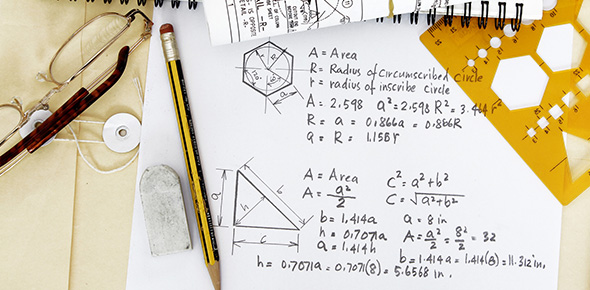# Finding Surface Area And Volume Of A Cylinder Quiz

6 Questions | Attempts: 1035SettingsBy completing this quiz you are going to be prepared for the final exam on surface area and volume of a cylinder.

• 1.
What is the formula for a cylinders surface area?
• A.

2*(r*r*h*3.14)+h*d*3.14

• B.

2*(r*r*3.14)+h*d*3.14

• C.

2*(r*r*3.14)*h*d*3.14

• D.

2*(r+r+3.14)+h*d+3.14

• 2.
What is the formula for a cylinders volume?
• A.

R+r*h*3.14

• B.

R*r*h+3.14

• C.

R*r+h+3.14

• D.

R*r*h*3.14

• 3.
What would be the volume if: diameter=9cm and the height 11cm?
• A.

623.14

• B.

434.39

• C.

329.63

• D.

699.80

• 4.
Is the circumference twice the diameter?
• A.

True

• B.

False

• 5.
What is the unit for surface area?
• A.

Units^2

• B.

Units^3

• C.

Units^3.14

• D.

Units^2.2

• 6.
To find the radius of a cylinder you should measure the diameter and divide by 2 ?
• A.

True

• B.

False

## Related TopicsBack to top
×

Wait!
Here's an interesting quiz for you.